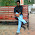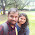# VOLTAGE TO FREQUENCY CONVERTER 9400

This section investigates the Teledyne 9400 series, which can be used as voltage to frequency or frequency to voltage converters. The series includes the 9400, 9401, 9402 converters. These converters have the same internal circuitry and connection diagrams, but they differ slightly in electrical characteristics. A complete V/F or F/V system can be formed simply by using two external capacitors, three resistors and a reference voltage. In addition, the 9400 series consist of CMOS and bipolar devices that can operate on dual or single supply voltages.

### VOLTAGE TO FREQUENCY CONVERTER

The 9400 is designed for pulse and square wave outputs having a frequency range of 1Hz to 100KHz. Furthermore, the input can be either current or voltage, and the output can interface with most forms of logic. The equivalent circuit consisting of an integrator, comparators, a delay network, a divide by 2 network and open collector output transistors. The input current is converted to a charge by the integrating capacitor and shows up as a linearly decreasing voltage at the output of the op amp integrator.

### CIRCUIT DIAGRAM OF VOLTAGE TO FREQUENCY CONVERTER USING 9400Voltage To Frequency Converter

### PIN OUT DIAGRAM OF TELEDYNE 9400 ICPin Out of 9400 IC

Pin 1: Ibias
A resistance Rbias is connected between pins 1 and 4. Specifications for 9400 are based on Rbias = 100kΩ ± 10%.

The circuit that can be used to reduce the output initially to zero is connected to this pin. The low frequency set point is determined by adjusting the voltage at this pin.

Pin 3: Iin
This pin is the negative input of the op amp, and the input current Iin is applied to it. Iin is 10µA for nominal full scale with an overrange current upto 50µA.

Pin 4: Vss

Pin 5: Vref out
When Cref is to be charged, the Cref charge/discharge control circuit connects this pin to the reference voltage Vref (Pin 7).

Pin 6: Ground

Pin7: Vref
A reference voltage from either  separate negative supply or the Vss may be applied to this pin.

Pin8: Pulse Frequency output
At this pin a pulse wave output is available whose frequency is proportional to the input voltage. Since this is an open collector output, it requires a pull up resistor and interface directly with all forms of logic.

Pin 9: Output Common
The emitters of both the output transistors are connected to this pin. A unipolar or bipolar output voltage swing can be obtained by connecting this pin either to ground or to the negative supply Vss, respectively. For high performance applications, the output ground (Pin 9) should be separated from the input ground (Pin 6).

Pin 10: Frequency Divide BY 2 Output.
This pin provides a square wave output that is one half the frequency of pulse output at pin 8. This output is an open collector and hence requires a pull up resistor. It is also compatible with all logic families.

Pin 11: Comparator Input
This pin is the positive input of the comparator and is connected to the output of the op-amp in the V/F mode. In the F/V mode, the input frequency is applied to the comparator input.

Pin 12: Amplifier Out
This is the output of the op-amp, which is a negative going ramp signal in the V/F mode. In the F/V mode, a voltage proportional to the frequency input is generated at this pin.

Pin 13: NC
No connection

Pin 14: VDD### Tittu

#### Author & Editor

I'm Tittu Thomas, An Electronics and Communication Engineer from India, Kerala. I love doing hobby electronics circuits, blogging, programming, etc. Started this blog while doing my B-Tech degree under CUSAT university.

1.Check out my blog
expaworld.blogspot.in
* cpu architecture
* pinouts
* programs
* modes of operations
* dsp processor

2.Sure...... And Thank You for the Comment.......

Like Us